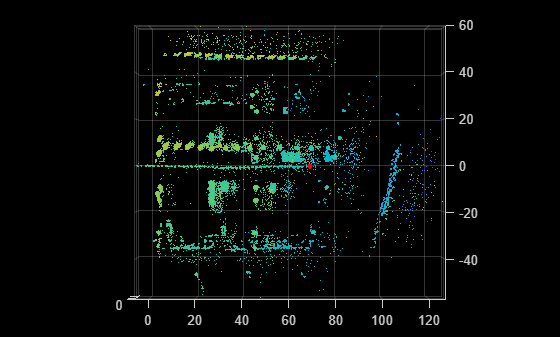# findPose

Localize point cloud within map using normal distributions transform (NDT) algorithm

Since R2021a

## Syntax

``currPose = findPose(ndtMap,ptCloud,initPose)``
``currPose = findPose(___,Name,Value)``

## Description

example

````currPose = findPose(ndtMap,ptCloud,initPose)` localizes the pose of the point cloud `ptCloud` within the NDT map `ndtMap` using the NDT algorithm. The function confines the search space to the submap, specified by the `SelectedSubmap` property of the `ndtMap` object.```
````currPose = findPose(___,Name,Value)` specifies options using one or more name-value arguments in addition to the input arguments in previous syntax. For example, `"MaxIterations",30` sets the maximum number of iterations before the function stops the NDT algorithm.```

## Examples

collapse all

Load a normal distributions transform (NDT) map from a MAT file.

```data = load('ndtMapParkingLot.mat'); ndtMap = data.ndtMapParkingLot;```

Load point cloud scans and pose estimates from a second MAT file.

```data = load('parkingLotData.mat'); ptCloudScans = data.parkingLotData.ptCloudScans; initPoseEsts = data.parkingLotData.initPoseEsts;```

Display the NDT map.

`show(ndtMap)`

Change the viewing angle to top-view.

`view(2)`

Select the submap centered around the first estimate.

```center = initPoseEsts(1).Translation; sz = [70 50 20]; ndtMap = selectSubmap(ndtMap,center,sz);```

Set the radius for visualization of the current location and the distance threshold to update the submap.

```radius = 0.5; distThresh = 15;```

Loop over the point clouds, localize them in the map, and update the selected submap as needed.

```numScans = numel(ptCloudScans); for n = 1:numScans ptCloud = ptCloudScans(n); initPose = initPoseEsts(n); poseTranslation = initPose.Translation; [isInside,distToEdge] = isInsideSubmap(ndtMap,poseTranslation); submapNeedsUpdate = ~isInside ... % Current pose is outside submap || any(distToEdge(1:2) < distThresh); % Current pose is close to submap edge if submapNeedsUpdate ndtMap = selectSubmap(ndtMap,poseTranslation,sz); end % Localize the point cloud scan in the map. currPose = findPose(ndtMap,ptCloud,initPose); % Display the position of the estimate as a circle. pos = [currPose.Translation(1:2) radius]; showShape('circle',pos,'Color','r'); % Pause to view the change. pause(0.05) end```## Input Arguments

collapse all

NDT map, specified as a `pcmapndt` object.

Point cloud in the sensor coordinate system, specified as a `pointCloud` object.

Initial estimate for the pose of the sensor in the map, specified as a `rigidtform3d` object.

### Name-Value Arguments

Specify optional pairs of arguments as `Name1=Value1,...,NameN=ValueN`, where `Name` is the argument name and `Value` is the corresponding value. Name-value arguments must appear after other arguments, but the order of the pairs does not matter.

Before R2021a, use commas to separate each name and value, and enclose `Name` in quotes.

Example: `"MaxIterations",30` stops the NDT algorithm after 30 iterations.

Expected percentage of outliers with respect to a normal distribution, specified as a scalar in the range [0, 1). The NDT algorithm assumes a point is generated by the mixture of a normal distribution for inliers and a uniform distribution for outliers. A larger value of `OutlierRatio` reduces the influence of outliers.

Data Types: `single` | `double`

Maximum number of iterations before the NDT algorithm stops, specified as a nonnegative integer.

Data Types: `single` | `double`

Tolerance between consecutive NDT iterations, specified as a 2-element vector with nonnegative values. The vector, [Tdiff Rdiff], represents the tolerance of absolute difference in translation and rotation, respectively, estimated in consecutive NDT iterations. Tdiff measures the Euclidean distance between two translation vectors. Rdiff measures the angular difference in degrees. The algorithm stops when the difference between estimated rigid transformations in the most recent consecutive iterations falls below the specified tolerance values.

Data Types: `single` | `double`

Display progress information, specified as a logical `0` (`false`) or `1` (`true`). Set `Verbose` to `true` to display progress information.

Data Types: `logical`

## Output Arguments

collapse all

Pose of the sensor in the map, returned as a `rigidtform3d` object. The function confines the search space to the submap, specified by the `SelectedSubmap` property of `ndtMap`.

## Tips

• To improve the accuracy and efficiency of localization, consider downsampling the point cloud using `pcdownsample` before using this function.

 Biber, P., and W. Strasser. “The Normal Distributions Transform: A New Approach to Laser Scan Matching.” In Proceedings 2003 IEEE/RSJ International Conference on Intelligent Robots and Systems (IROS 2003) (Cat. No.03CH37453) Vol. 3, 2743–48. Las Vegas, Nevada, USA: IEEE, 2003. https://doi.org/10.1109/IROS.2003.1249285.

 Magnusson, Martin. "The Three-Dimensional Normal-Distributions Transform: An Efficient Representation for Registration, Surface Analysis, and Loop Detection." PhD thesis, Örebro universitet, 2009. http://urn.kb.se/resolve?urn=urn:nbn:se:oru:diva-8458 urn:nbn:se:oru:diva-8458.